Open In App

# Design asynchronous Up/Down counter

Prerequisite : Ripple counter.

In asynchronous/ripple counter output of the first flip-flop is provided as the clock to the second flip-flop i.e flip-flop(FF) are not clocked simultaneously.
Circuit is simpler, but speed is slow.

Asynchronous counter basics :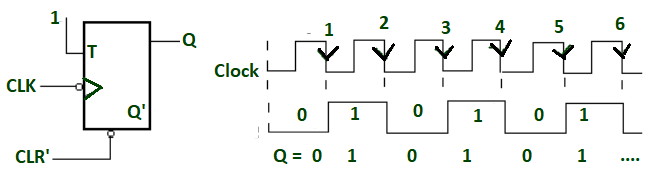1 bit asynchronous/ripple counter

When -ve edge clock pulse is applied and input is given to FF logic 1 then the output state of FF will toggle for every falling edge. The output frequency will be f/2 (If f is clock frequency). It is known as binary or mod -2 counter or bit ripple counter.
It has 2 unique output states (0 and 1).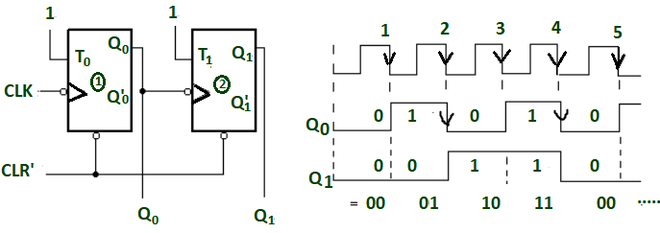2 bit asynchronous Up counter.

When two FFs are connected in series and output of one FF is act as clock for 2nd FF. So the state of 2nd FF will change only when output and 1st FF is logic 1 and falling edge occur. The output frequency of Q1 is f/4(if f is clock frequency).
It can generate 4 different unique states. This is known as divide by 4 circuits or mod 4 ripple counter.
Here output is taken as Q1(MSB) Q0(LSB).

By this, we can conclude that –

If there are n FFs then the output frequency will be divide by 2n. Also generate 2n unique states.

So the frequency division basically forms counting state.
Here we are seeing that the output of the 1st FF act as clock for 2nd FF. Suppose the FF takes 30ns for generating output(i.e. propagation delay because of gates). Therefore, the output of second FF will be obtained after 60 ns. So the propagation delay is ripples through the FFs and becomes more when the number of FFs increases.
Therefore, asynchronous counter are too slow for generating big counting.

• As we know, when the output state (i.e. Q) of previous FF is feed as clock to next FF then the counter will perform up counting as you seen above(i.e. 0 1 2 3).
After 4th -ve edge clock pulse the sequence will repeat.
• When the complemented output state (i.e. Q’) of previous FF is feed as clock to next FF then the counter will perform down counting as you seen below(i.e. 3 2 1 0).
After 4th -ve edge clock pulse the sequence will repeat.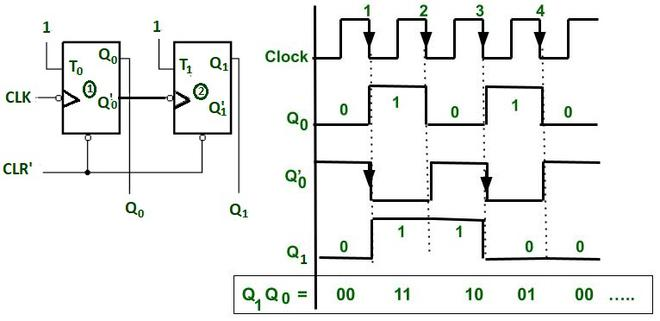2 bit asynchronous down counter

Now we are designing Up/Down counter.
Up/Down counter is the combination of both the counters in which we can perform up or down counting by changing the Mode control input.

Design of 3 bit Asynchronous up/down counter
It is used more than separate up or down counter.

1. In this a mode control input (say M) is used for selecting up and down mode.
2. A combinational circuit is required between each pair of flip-flop to decide whether to do up or do down counting.

For n = 3, i.e for 3 bit counter –
Maximum count = 2n -1 and number of states are 2n.

Steps involve in design are :
Step 1 : Decision for Mode control input –Decision for mode control input

When M = 0, then Y= Q, therefore it will perform Up counting (As discussed above).
When M = 1, then Y= Q’ therefore it will perform Down counting (As discussed above).
Combinational circuit is required for deciding mode control(i.e whether counter will perform Up counting or Down counting).
So the all possible combinations are –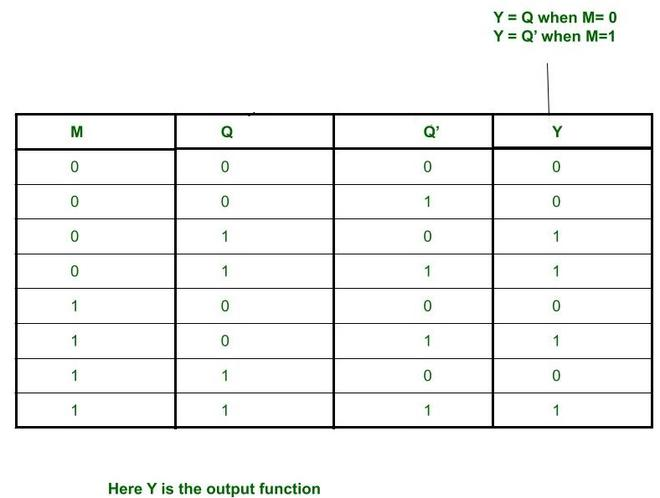K-map for finding output Y that will be given as clock to next FF.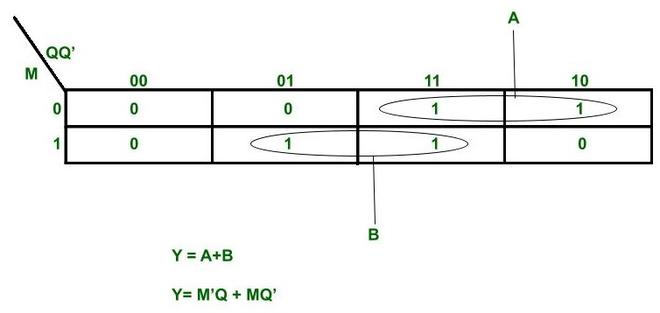K map for finding Y

Step 2 : Insertion of Combinational logic between every pair of FFs –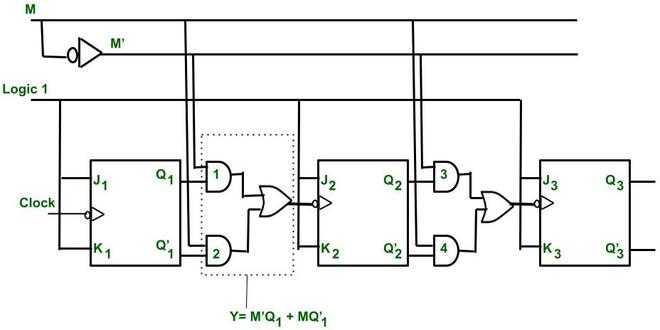Up/Down Counter

Timing diagram :
Initially Q3 = 0, Q2 = 0, Q1 = 0.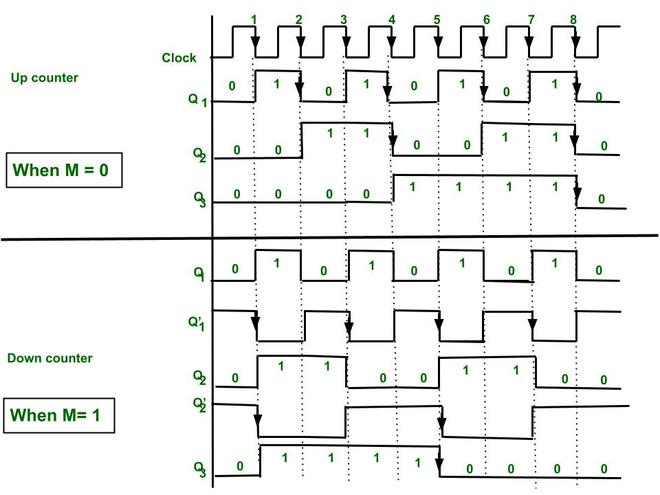Timing diagram for 3 bit asynchronous up/down counter

Case 1 – When M=0, then M’ =1.
Put this in Y= M’Q + MQ’= Q So Q is acting as clock for next FFs.
Therefore, the counter will act as Up counter.

Explanation of Up counter –

• The 1st FF is connected to logic 1. Therefore, it will toggle for every falling edge.
• The 2nd FF input is connected to Q1.Therefore it changes its state when Q1= 1 and there is falling edge of clock.
• Similarly, 3rd FF is connected to Q2. Therefore, it changes its state when Q2= 1 and there is falling edge of clock.
• By this we can generate counting states of Up counter.
• After every 8th falling edge the counter is again reaching to state 0 0 0.
Therefore, it is also known as divide by 8 circuit or mod 8 counter.

Case 2 – When M=1, then M’ =0.
Put this in Y= M’Q + MQ’= Q’.  So Q’ is acting as clock for next FFs.
Therefore, the counter will act as Down counter.

Explanation of Down counter –

• The 1st FF is connected to logic 1. Therefore, it will toggle for every falling edge.
• The 2nd FF input is connected to Q’1.Therefore it changes its state when Q’1= 1 and there is falling edge of clock.
• Similarly, 3rd FF is connected to Q’2. Therefore, it changes its state when Q’2= 1 and there is falling edge of clock.
• By this we can generate counting states of down counter.
• After every 8th falling edge the counter is again reaching to state 0 0 0.
Therefore, it is also known as divide by 8 circuit or mod 8 counter.